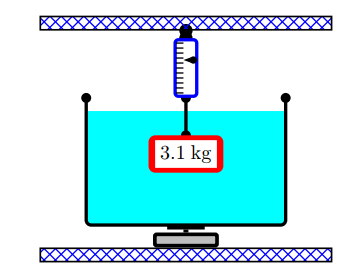# Problem: A beaker of mass 1 kg containing 2.5 kg of water rests on a scale. A 3.1 kg block of a metallic alloy of density 4900 kg/m3 is suspended from a spring scale and is submerged in the water of density 1000 kg/m3 as shown in the figure. What does the hanging scale read? The acceleration due to gravity is 9.8 m/s 2. A. 21.3715 B. 16.043 C. 23.0577 D. 28.3774 E. 14.3733 F. 31.6615 G. 27.8826 H. 24.18 I. 23.8994 J. 27.6033

###### FREE Expert Solution
88% (379 ratings)
###### Problem Details

A beaker of mass 1 kg containing 2.5 kg of water rests on a scale. A 3.1 kg block of a metallic alloy of density 4900 kg/m3 is suspended from a spring scale and is submerged in the water of density 1000 kg/m3 as shown in the figure.

What does the hanging scale read? The acceleration due to gravity is 9.8 m/s 2.

A. 21.3715

B. 16.043

C. 23.0577

D. 28.3774

E. 14.3733

F. 31.6615

G. 27.8826

H. 24.18

I. 23.8994

J. 27.6033